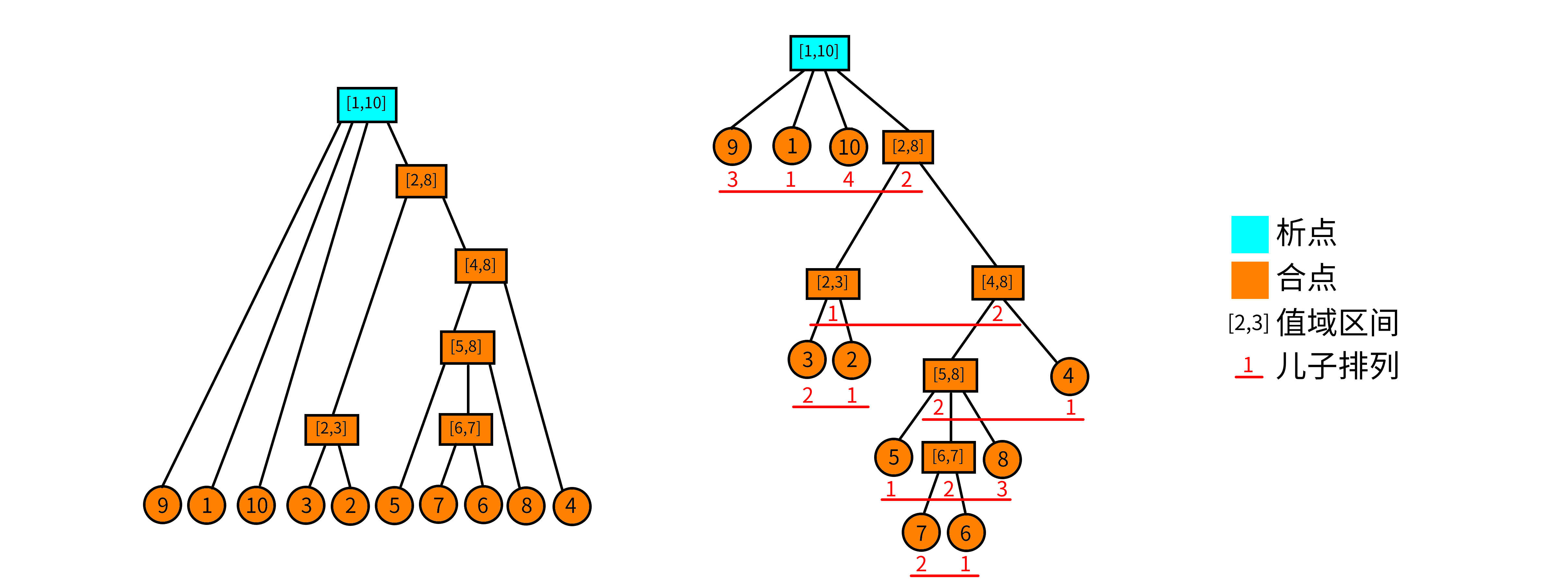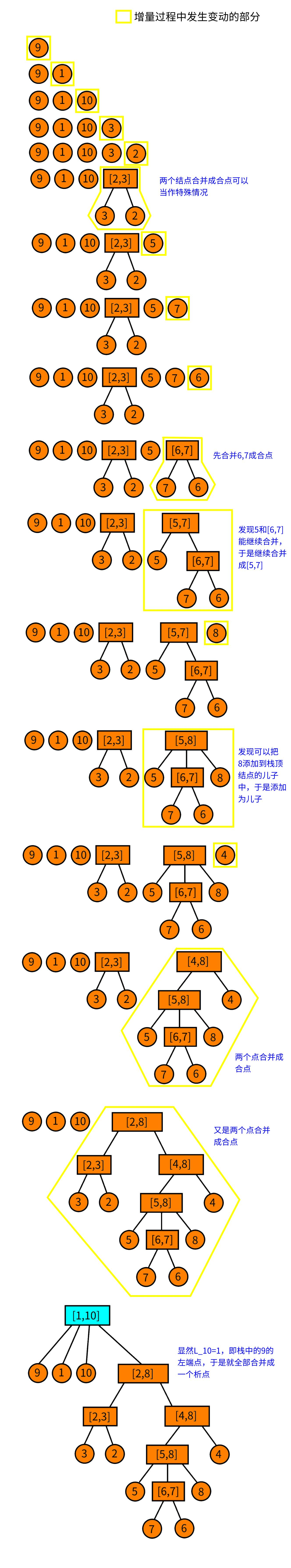# 析合树

• 如果$1\leq a\leq l\leq b\leq r\leq n$，且$(P,[a,b])$和$(P,[l,r])$都是连续段，那么它们的交集$(P,[l,b])$，并集$(P,[a,r])$，差集$(P,[a,l))$和$(P,(b,r])$都是连续段。

• 本原段：一个段是本原段，当且仅当不存在另外一个连续段与其部分相交。

• 合点：如果一个顶点的儿子排列是递增或递减的，则这个顶点为合点。
• 析点：一个顶点是析点当且仅当这个顶点不是合点1. 如果$y$是一个合点且拥有至少两个孩子，且$x$可以作为$y$的新儿子，那么将$x$加入到$y$的儿子序列中，之后$y$出栈并令$x=y$。
2. 如果第1步没有成立，则尝试创建一个新合点$z$，它的孩子为$y$和$x$，之后将$y$出栈并令$x=z$。
3. 如果前面几步都不成立，创建一个析点$z$，并将$x$和栈顶部尽可能少的顶点作为$z$的孩子并出栈，最后令$x=z$。
4. 如果前面几步都不成立，将$x$入栈，并结束流程。